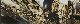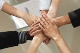# GridCalculatedColumn

GridTop achievementsRank 2
3ms asked on 27 Oct 2008, 02:26 AM
I created a nested grid using the example programmatically from here.

I want to add a Calculated Column to a radgrid and added the following code but am unsure how to define the datafields and expression.

calculatedColumn = new GridCalculatedColumn();
calculatedColumn.DataFormatString = "{0:C}";

## 6 Answers, 1 is accepted

0
AcceptedPrincy
Top achievementsRank 2
answered on 27 Oct 2008, 05:22 AM
Hello,

Try setting the DataFields and the Expression attributes for the Calculated column as shown below:
cs:

Thanks
Princy.
03ms
Top achievementsRank 2
answered on 27 Oct 2008, 03:02 PM
Princy,

Thanks for your help.  that got my columns working, now the formatting does not work.  Is there something I need to do other than what is here?

calculatedColumn = new GridCalculatedColumn();
calculatedColumn.DataFields = new string[] {"AmountBilled", "AmountReceived"};
calculatedColumn.Expression = "{0}-{1}";
calculatedColumn.DataFormatString = "{0:c2}";

Thanks,

3ms
0
AcceptedPrincy
Top achievementsRank 2
answered on 28 Oct 2008, 05:49 AM
Hello,

You have to set the DataType for the column so as to get the DataFormatString applied to the column. Check out the code below to find out how to get this done.
cs:
 GridCalculatedColumn calcol = new GridCalculatedColumn(); calcol.DataFields =new string[]{"AmountBilled","AmountReceived"}; calcol.Expression ="{0}-{1}"; calcol.DataFormatString = "{0:c2}"; calcol.DataType = System.Type.GetType("System.Double"); tableViewProjects.Columns.Add(calcol);

Thanks
Princy.
0Greg
Top achievementsRank 1
answered on 14 Nov 2008, 03:28 PM
Is there any way to use something like a CalculatedColumn to calculate a value from code-behind...

Something like

C#:
CalculatedColumn value = getColValue(Column1 Value)

 private string getColValue(string inputVal) { //Calcuations - returns a string }

Shot.
Greg

0Yavor
Telerik teamanswered on 17 Nov 2008, 06:41 AM
Hello Greg,

You can use code-behind, to add the column dinamically, and set its properties and to specify which columns its value will be based on. Other than that, you can use code-behind to alter the value dynamically, by accessing the relevant cell.

All the best,
Yavor
the Telerik team

Check out Telerik Trainer, the state of the art learning tool for Telerik products.
03ms
Top achievementsRank 2
answered on 09 Dec 2008, 09:29 PM
Princy,

Thanks for your help!  I added the following code to show the aggregate in the footer and I'm getting the error, "Sum is not supported for type System.Object".  This is all in the first detail table of my grid.  All is done on Page Load.

Unit.Percentage(98);

true;

true;

GridPagerMode.NextPrevAndNumeric;

false;

true;

true;

boundColumn =

new GridBoundColumn();

boundColumn.DataField =

"ContractAmount";

"Contract Amount";

boundColumn.DataFormatString =

"{0:C}";

boundColumn.Aggregate =

GridAggregateFunction.Sum;

Tags
Grid3ms
Top achievementsRank 2Princy
Top achievementsRank 23ms
Top achievementsRank 2Greg
Top achievementsRank 1Yavor
Telerik team